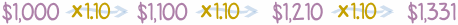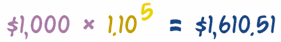# 复利：定期复利

## 复利简介（去这里了解更多）## 公式### 例子：投资 ￥1,000，5年，年利率 10%：

PV × (1 + r)n = FV
￥1,000 × (1 + 0.10)5 = FV
￥1,000 × 1.105 = ￥1,610.51

### 例子：投资 ￥1,000，5年，年利率6%：

PV × (1 + r)n = FV
￥1,000 × (1 + 0.06)5 = FV
￥1,000 × 1.065 = ￥1,338.23

## （一年内）

……但一年里计算多次利息，每次的利息都加到本金上……

……所以一年也有复利计算.

• 上半年 5%，
• 下半年 5% ，10%，半年复利

### 两个年利率？

 例子 10% 名义利率（声明的利率） 10.25% 有效年利率（计算复利后的利率） 有效年利率是实际的利率！

### 算法

• 声明的利率（名义利率 "r"）
• 计算复利的次数（"n"）。

FV = PV (1+r)n

r / n

FV = PV (1+(r/n))n

PV = 现值
r = 年利率
n = 期数

FV = ￥1,000 (1+(0.10/2))2 = ￥1,000(1.05)2 = ￥1,000 × 1.1025 = ￥1,102.50

(1+(r/n))n = (1.05)2 = 1.1025

(1+(r/n))n − 1 = 0.1025 = 10.25%

### 例子：广告上写的是： "月复利 6%"，实际有效年利率是多少？

r = 0.06（6% 的小数）
n = 12

= (1+(0.06/12))12 − 1

= (1.005)12 − 1 = 0.06168 = 6.168%

### 例子：7% 年利率，一年计算 4次复利。

r = 0.07（7% 的小数）
n = 4

FV = PV (1+(0.07/4))4

FV = PV (1+(0.07/4))4

FV = PV (1.0719……)

r / n 把年利率分开为 "n"期 计算 "n" 次复利： 不要忘了减掉 "1"

## 数值表

1   1.00% 5.00% 10.00% 20.00% 100.00%

4   1.00% 5.09% 10.38% 21.55% 144.14%
12   1.00% 5.12% 10.47% 21.94% 161.30%
365   1.01% 5.13% 10.52% 22.13% 171.46%
... ……

### 连续？## 应用

### 例子：￥10,000，连续复利 2年，8%

FV = PV × (1+r)n

FV = ￥10,000 × (1+0.08329)2

FV = ￥10,000 × 1.173511... = ￥11,735.11

## 结论

 有效年利率 = (1+(r/n))n − 1 其中： r = 名义利率（声明的利率） n = 计算复利期数（例子：月复利=12）